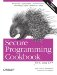# Parsing a String Containing a Number in Scientific Notation

Problem

You have a string containing a number in scientific notation, and you want to store the number's value in a double variable.

Solution

The most direct way to parse a scientific notation number is by using the C++ library's built-in stringstream class declared in , as you can see in Example 3-7.

Example 3-7. Parsing a number in scientific notation

```#include
#include
#include

using namespace std;

double sciToDub(const string& str) {

stringstream ss(str);
double d = 0;
ss >> d;

if (ss.fail( )) {
string s = "Unable to format ";
s += str;
s += " as a number!";
throw (s);
}

return (d);
}

int main( ) {

try {
cout << sciToDub("1.234e5") << endl;
cout << sciToDub("6.02e-2") << endl;
cout << sciToDub("asdf") << endl;
}
catch (string& e) {
cerr << "Whoops: " << e << endl;
}
}```

Following is the output from this code:

```123400
0.0602
Whoops: Unable to format asdf as a number!```

Discussion

The stringstream class is, not surprisingly, a string that behaves like a stream. It is declared in . If you need to parse a string that contains a number in scientific notation (see also Recipe 3.2), a stringstream will do the job nicely. The standard stream classes already "know" how to parse numbers, so don't waste your time reimplementing this logic if you don't have to.

In Example 3-7, I wrote the simple function sciToDub that takes a string parameter and returns the double it contains, if it is valid. Within sciToDub, I use the stringstream as follows:

```stringstream ss(str); // Construct from a string
double d = 0;
ss >> d;

if (ss.fail( )) {
string s = "Unable to format ";
s += str;
s += " as a number!";
throw (s);
}
return (d);```

The most important part here is that all you have to do is use the right-shift operator (>>) to read from the string stream into a double, just as you would read from cin.

Well, that's not all you have to do. If there's a value in the stringstream that can't be written to the variable on the right side of the >> operator, the fail bit is set on the stream. You can check this bit using the fail member function (this is actually a member function of basic_ios, which is a superclass of stringstream). Additionally, the variable on the righthand side of the >> operator is unchanged if the operation fails.

In the interest of being generic, however, you can avoid having to write separate versions of sciToDub for ints, floats, doubles, and whatever else you want to convert to by writing a function template. Consider this new version:

```template
T strToNum(const string& str) {
stringstream ss(str);
T tmp;
ss >> tmp;

if (ss.fail( )) {
string s = "Unable to format ";
s += str;
s += " as a number!";
throw (s);
}

return (tmp);
}```

Now, if you want to convert a string to a numeric type, you can do it like this:

```double d = strToNum("7.0");
float f = strToNum("7.0");
int i = strToNum("7.0");```

You can also make the type of character a template parameter, but that's straightforward to do, so I'll leave it as an exercise for you.

Recipe 3.2Secure Programming Cookbook for C and C++: Recipes for Cryptography, Authentication, Input Validation & More
ISBN: 0596003943
EAN: 2147483647
Year: 2006
Pages: 241